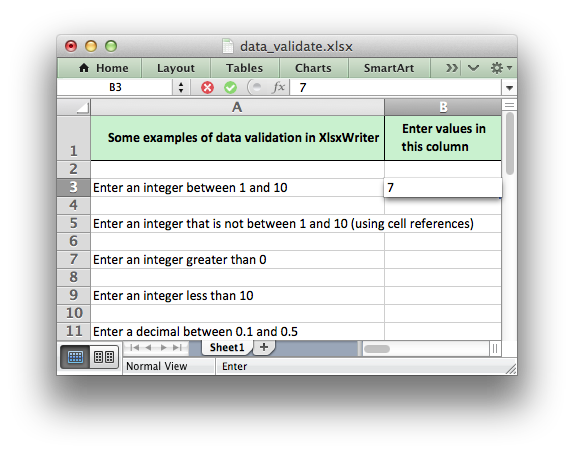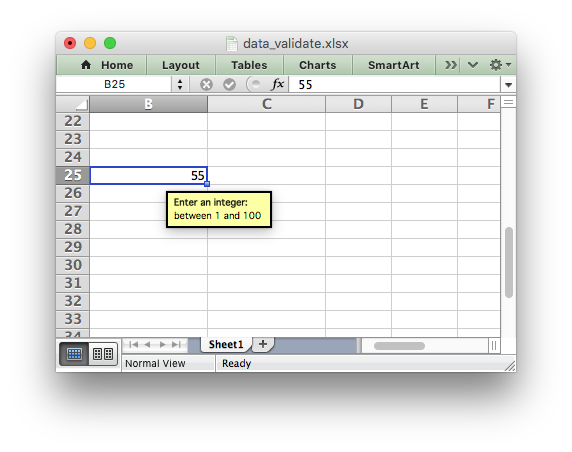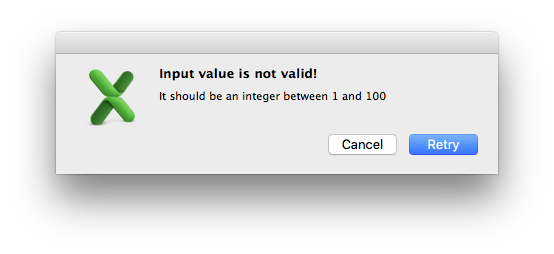libxlsxwriter
Working with Data Validation

Data validation is a feature of Excel which allows restrictions to be placed on the data that a user enters in a cell and to display associated help and warning messages. It can also be used to restrict input to values in a drop down list.

A typical use case might be to restrict data in a cell to integer values in a certain range, to provide a help message to indicate the required value and to issue a warning if the input data doesn't meet the stated criteria. In `libxlsxwriter` this can be done as follows:

lxw_data_validation *data_validation = calloc(1, sizeof(lxw_data_validation));
data_validation->minimum_number = 1;
data_validation->maximum_number = 10;
worksheet_data_validation_cell(worksheet, CELL("B3"), data_validation);If the user inputs a value that doesn't match the specified criteria an error message is displayed:For more information on data validation see the Microsoft support article Description and examples of data validation in Excel.

The following sections describe how to use the `worksheet_data_validation_cell()` and `worksheet_data_validation_range()` functions and the various options of lxw_data_validation.

# The data validation functions

The `worksheet_data_validation_cell()` and `worksheet_data_validation_range()` functions are used to construct an Excel data validation.

The data validation can be applied to a single cell or a range of cells:

worksheet_data_validation_cell( worksheet, 2, 1, data_validation);
worksheet_data_validation_range(worksheet, 2, 1, 4, 1, data_validation);
// Same as above using the CELL() and RANGE() macros.
worksheet_data_validation_cell( worksheet, CELL("B3"), data_validation);
worksheet_data_validation_range(worksheet, RANGE("B3:B5"), data_validation);

The lxw_data_validation struct used in these functions is explained below.

# The lxw_data_validation struct

The lxw_data_validation struct is used to set the properties of a data validation. A typical usage would look something like this:

lxw_data_validation *data_validation = calloc(1, sizeof(lxw_data_validation));
data_validation->value_number = 10;
data_validation->show_input = LXW_VALIDATION_OFF;

This example shows the main properties of lxw_data_validation and Excel data validations:

• `validate`: The type of data to restrict the validation to. In this case it is whole numbers.
• `criteria`: The criteria by which the data will be evaluated. In this case whether the input is greater than a value.
• `value`: The value that the criteria applies to. This has several different versions for different types of data. This could also be a `minimum` and `maximum` value if the criteria used is a "BETWEEN" criteria. This is explained in the value, minimum, maximum section below.
• Other options such as `show_input` or `input_message`. These parameters are explained in the Data validation options section.

Note, in the examples in this document the `data_validation` variable is shown as dynamically allocated, however an address of a static or automatic variable could also be used. In these cases make sure that the struct members are initialized to zero before setting other parameters.

## validate

The `validate` parameter is used to set the type of data that you wish to validate:

It is always required and it has no default value. In Excel the validate parameters are:

• Whole
• Decimal
• List
• Date
• Time
• Text Length
• Custom
• Any

The equivalent parameters in libxlsxwriter are defined in lxw_validation_types:

## criteria

The `criteria` parameter is used to set the criteria by which the data in the cell is validated. It is almost always required except for the `list`, `custom` and `any` validate options. It has no default value:

Allowable values are defined in lxw_validation_criteria:

The `list`, `custom` and `any` validate options don't require a `criteria`. If you specify one it will be ignored.

## value, minimum, maximum

The `value` parameters are used to set the limiting value to which the `criteria` is applied. It is always required and it has no default value. There are different types of `value` parameter associated with different types of data. They are:

`value_number`:

The `value_number` parameter is used to set the limiting value to which the criteria is applied using a whole or decimal number. It is typically used with LXW_VALIDATION_TYPE_INTEGER and LXW_VALIDATION_TYPE_DECIMAL and LXW_VALIDATION_TYPE_LENGTH.

data_validation->value_number = 0;
data_validation->value_number = 10.5;
data_validation->value_number = 3;

`value_formula`:

The `value_formula` parameter is used to set the limiting value to which the criteria is applied using a cell reference. It is valid for any of the lxw_validation_types types that end in `_FORMULA`:

data_validation->value_formula = "=E3";
data_validation->criteria = LXW_VALIDATION_CRITERIA_EQUAL;
data_validation->value_formula = "=H1";
data_validation->value_formula = "=\$E\$4:\$G\$4";

`value_list`:

The `value_list` parameter is used to set a list of strings for a drop down list. The list should be a `NULL` terminated array of char* strings:

char *list[] = {"open", "high", "close", NULL};
data_validation->value_list = list;

Note, when using the LXW_VALIDATION_TYPE_LIST validation with a list of strings, like in the last example above, Excel stores the strings internally as a Comma Separated Variable string. The total length for this string, including commas, cannot exceed the Excel limit of 255 characters. For longer sets of data you should use a range reference like the previous example above.

`value_datetime`:

The `value_datetime` parameter is used to set the limiting value to which the LXW_VALIDATION_TYPE_DATE or LXW_VALIDATION_TYPE_TIME criteria is applied using a lxw_datetime struct:

lxw_datetime datetime1 = {2017, 9, 24, 0, 0, 0};
lxw_datetime datetime2 = { 0, 0, 0, 12, 30, 0};
data_validation->criteria = LXW_VALIDATION_CRITERIA_EQUAL;
data_validation->value_datetime = datetime1;
data_validation->criteria = LXW_VALIDATION_CRITERIA_EQUAL;
data_validation->value_datetime = datetime2;

The `minimum` and `maximum` parameters are used to set the lower and upper limiting values when the `criteria` is either LXW_VALIDATION_CRITERIA_BETWEEN or LXW_VALIDATION_CRITERIA_NOT_BETWEEN. The parameters are:

• `minimum_number`
• `minimum_formula`
• `minimum_datetime`
• `maximum_number`
• `maximum_formula`
• `maximum_datetime`

They are similar to the `value` parameters described above. For example:

data_validation->minimum_number = 1;
data_validation->maximum_number = 10;
data_validation->minimum_formula = "=E3";
data_validation->maximum_formula = "=F3";
data_validation->minimum_datetime = datetime1;
data_validation->maximum_datetime = datetime2;

# Data validation options

## ignore_blank

The `ignore_blank` parameter is used to toggle on and off the 'Ignore blank' option in the Excel data validation dialog. When the option is on the data validation is not applied to blank data in the cell. It is on by default:

data_validation->minimum_number = 1;
data_validation->maximum_number = 10;
data_validation->ignore_blank = LXW_VALIDATION_OFF;

## dropdown

The `dropdown` parameter is used to toggle on and off the 'In-cell dropdown' option in the Excel data validation dialog. When the option is on a dropdown list will be shown for `list` validations. It is on by default.

data_validation->value_list = list;
data_validation->dropdown = LXW_VALIDATION_OFF;

## input_title

The `input_title` parameter is used to set the title of the input message that is displayed when a cell is entered. It has no default value and is only displayed if the input message is also displayed. See the `input_message` parameter below.

The maximum title length is 32 characters.

## input_message

The `input_message` parameter is used to set the input message that is displayed when a cell is entered. It has no default value:

data_validation->minimum_number = 1;
data_validation->maximum_number = 100;
data_validation->input_title = "Enter an integer:";
data_validation->input_message = "between 1 and 100";

The input message generated from the above example is:The message can be split over several lines using newlines. The maximum message length is 255 characters.

## show_input

The `show_input` parameter is used to toggle on and off the 'Show input message when cell is selected' option in the Excel data validation dialog. When the option is off an input message is not displayed even if it has been set using `input_message`. It is on by default.

## error_title

The `error_title` parameter is used to set the title of the error message that is displayed when the data validation criteria is not met. The default error title is 'Microsoft Excel'. The maximum title length is 32 characters.

## error_message

The `error_message` parameter is used to set the error message that is displayed when a cell is entered. The default error message is "The value you entered is not valid. A user has restricted values that can be entered into the cell". A non-default error message can be displayed as follows:

data_validation->minimum_number = 1;
data_validation->maximum_number = 100;
data_validation->input_title = "Enter an integer:";
data_validation->input_message = "between 1 and 100";
data_validation->error_title = "Input value is not valid!";
data_validation->error_message = "It should be an integer between 1 and 100";

Which give the following message:The message can be split over several lines using newlines. The maximum message length is 255 characters.

## error_type

The `error_type` parameter is used to specify the type of error dialog that is displayed. There are 3 lxw_validation_error_types options:

## show_error

The `show_error` parameter is used to toggle on and off the 'Show error alert after invalid data is entered' option in the Excel data validation dialog. When the option is off an error message is not displayed even if it has been set using `error_message`. It is on by default.

# Examples

// Restrict input to an integer between 1 and 10.
data_validation->minimum_number = 1;
data_validation->maximum_number = 10;
// Restrict input to an integer not between 1 and 10 (using cell references).
data_validation->minimum_formula = "=E3";
data_validation->maximum_formula = "=F3";
// Restrict input to a decimal between 0.1 and 0.5.
data_validation->minimum_number = 0.1;
data_validation->maximum_number = 0.5;
// Select a value from a drop down list.
char *list[] = {"open", "high", "close", NULL};
data_validation->value_list = list;
// Select a value from a drop down list (using a cell range).
data_validation->value_formula = "=\$E\$4:\$G\$4";
// Restrict input to a date between 1/1/2008 and 12/12/2008.
lxw_datetime datetime1 = {2008, 1, 1, 0, 0, 0};
lxw_datetime datetime2 = {2008, 12, 12, 0, 0, 0};
data_validation->minimum_datetime = datetime1;
data_validation->maximum_datetime = datetime2;
// Restrict input to a string longer than 3 characters.
data_validation->value_number = 3;
// Restrict input to a value if a formula is true.
data_validation->value_formula = "=AND(F5=50,G5=60)";
// Display a custom info message when integer isn't between 1 and 100.
data_validation->minimum_number = 1;
data_validation->maximum_number = 100;
data_validation->input_title = "Enter an integer:";
data_validation->input_message = "between 1 and 100";
data_validation->error_title = "Input value is not valid!";
data_validation->error_message = "It should be an integer between 1 and 100";

For a full example see data_validate.c.

lxw_data_validation::value_formula
char * value_formula
Definition: worksheet.h:982
lxw_data_validation::criteria
uint8_t criteria
Definition: worksheet.h:931
lxw_data_validation::input_message
char * input_message
Definition: worksheet.h:1062
lxw_data_validation::input_title
char * input_title
Definition: worksheet.h:1053
LXW_VALIDATION_TYPE_TIME_FORMULA
@ LXW_VALIDATION_TYPE_TIME_FORMULA
Definition: worksheet.h:150
lxw_data_validation
Worksheet data validation options.
Definition: worksheet.h:920
lxw_data_validation::minimum_datetime
lxw_datetime minimum_datetime
Definition: worksheet.h:1025
lxw_data_validation::ignore_blank
uint8_t ignore_blank
Definition: worksheet.h:937
lxw_data_validation::value_datetime
lxw_datetime value_datetime
Definition: worksheet.h:1007
lxw_data_validation::error_title
char * error_title
Definition: worksheet.h:1070
LXW_VALIDATION_TYPE_LENGTH
@ LXW_VALIDATION_TYPE_LENGTH
Definition: worksheet.h:158
lxw_data_validation::minimum_number
double minimum_number
Definition: worksheet.h:1013
LXW_VALIDATION_TYPE_INTEGER_FORMULA
@ LXW_VALIDATION_TYPE_INTEGER_FORMULA
Definition: worksheet.h:120
lxw_data_validation::maximum_number
double maximum_number
Definition: worksheet.h:1031
lxw_data_validation::value_list
char ** value_list
Definition: worksheet.h:1001
LXW_VALIDATION_TYPE_DECIMAL
@ LXW_VALIDATION_TYPE_DECIMAL
Definition: worksheet.h:123
RANGE
#define RANGE(range)
Convert an Excel A1:B2 range into a (first_row, first_col, last_row, last_col) sequence.
Definition: utility.h:82
LXW_VALIDATION_CRITERIA_GREATER_THAN
@ LXW_VALIDATION_CRITERIA_GREATER_THAN
Definition: worksheet.h:189
lxw_data_validation::maximum_formula
char * maximum_formula
Definition: worksheet.h:1037
LXW_VALIDATION_TYPE_CUSTOM_FORMULA
@ LXW_VALIDATION_TYPE_CUSTOM_FORMULA
Definition: worksheet.h:166
LXW_VALIDATION_TYPE_TIME
@ LXW_VALIDATION_TYPE_TIME
Definition: worksheet.h:147
lxw_datetime
Struct to represent a date and time in Excel.
Definition: common.h:155
LXW_VALIDATION_TYPE_LIST
@ LXW_VALIDATION_TYPE_LIST
Definition: worksheet.h:130
lxw_data_validation::show_input
uint8_t show_input
Definition: worksheet.h:946
LXW_VALIDATION_TYPE_INTEGER
@ LXW_VALIDATION_TYPE_INTEGER
Definition: worksheet.h:116
lxw_data_validation::validate
uint8_t validate
Definition: worksheet.h:925
LXW_VALIDATION_OFF
@ LXW_VALIDATION_OFF
Definition: worksheet.h:104
LXW_VALIDATION_TYPE_LIST_FORMULA
@ LXW_VALIDATION_TYPE_LIST_FORMULA
Definition: worksheet.h:134
lxw_data_validation::minimum_formula
char * minimum_formula
Definition: worksheet.h:1019
lxw_data_validation::value_number
double value_number
Definition: worksheet.h:975
lxw_data_validation::error_message
char * error_message
Definition: worksheet.h:1081
lxw_data_validation::dropdown
uint8_t dropdown
Definition: worksheet.h:969
worksheet_data_validation_range
lxw_error worksheet_data_validation_range(lxw_worksheet *worksheet, lxw_row_t first_row, lxw_col_t first_col, lxw_row_t last_row, lxw_col_t last_col, lxw_data_validation *validation)
Add a data validation to a range.
worksheet_data_validation_cell
lxw_error worksheet_data_validation_cell(lxw_worksheet *worksheet, lxw_row_t row, lxw_col_t col, lxw_data_validation *validation)
Add a data validation to a cell.
LXW_VALIDATION_TYPE_DATE
@ LXW_VALIDATION_TYPE_DATE
Definition: worksheet.h:137
LXW_VALIDATION_CRITERIA_NOT_BETWEEN
@ LXW_VALIDATION_CRITERIA_NOT_BETWEEN
Definition: worksheet.h:180
CELL
#define CELL(cell)
Convert an Excel A1 cell string into a (row, col) pair.
Definition: utility.h:45
lxw_data_validation::maximum_datetime
lxw_datetime maximum_datetime
Definition: worksheet.h:1043
LXW_VALIDATION_CRITERIA_BETWEEN
@ LXW_VALIDATION_CRITERIA_BETWEEN
Definition: worksheet.h:177